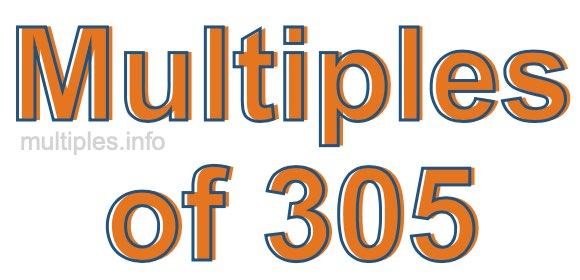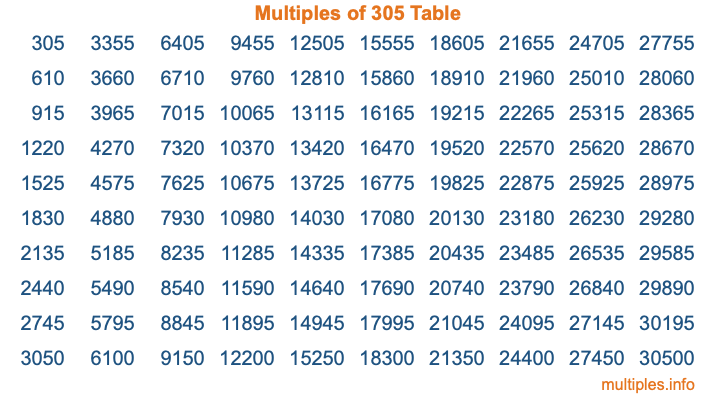Multiples of 305Welcome to the Multiples of 305 page. Here we will first teach you everything you will ever need to know about the multiples of 305, and then give you a study guide summary of everything we taught you to make sure you remember it all. Use this page to look up facts and learn information about the multiples of 305. This page will make you a multiples of three hundred five expert!

Definition of Multiples of 305
Multiples of 305 are all the numbers that when divided by 305 equal an integer. Each of the multiples of 305 are called a multiple. A multiple of 305 is created by multiplying 305 by an integer.

Therefore, to create a list of multiples of 305, you start with 1 multiplied by 305, then 2 multiplied by 305, then 3 multiplied by 305, and so on for as long as you want. Thus, the list of the first five multiples of 305 is 305, 610, 915, 1220, and 1525. To see a larger list of multiples of 305, see the printable image of Multiples of 305 further down on this page. We also have a category where you can choose any nth multiple of 305.

Multiples of 305 Checker
The Multiples of 305 Checker below checks to see if any number of your choice is a multiple of 305. In other words, it checks to see if there is any number (integer) that when multiplied by 305 will equal your number. To do that, we divide your number by 305. If the the quotient is an integer, then your number is a multiple of 305.

Is  a multiple of 305?

Least Common Multiple of 305 and ...
A Least Common Multiple (LCM) is the lowest multiple that two or more numbers have in common. This is also called the smallest common multiple or lowest common multiple and is useful to know when you are adding our subtracting fractions. Enter one or more numbers below (305 is already entered) to find the LCM.

Check out our LCM Calculator if you need more details about the Least Common Multiple or if you need the LCM for different numbers for adding and subtraction fractions.

nth Multiple of 305
As we stated above, 305 is the first multiple of 305, 610 is the second multiple of 305, 915 is the third multiple of 305, and so on. Enter a number below to find the nth multiple of 305.

th multiple of 305

Multiples of 305 vs Factors of 305
305 is a multiple of 305 and a factor of 305, but that is where the similarities end. All postive multiples of 305 are 305 or greater than 305. All positive factors of 305 are 305 or less than 305.

Below is the beginning list of multiples of 305 and the factors of 305 so you can compare:

Multiples of 305: 305, 610, 915, 1220, 1525, etc.

Factors of 305: 1, 5, 61, 305

As you can see, the multiples of 305 are all the numbers that you can divide by 305 to get a whole number. The factors of 305, on the other hand, are all the whole numbers that you can multiply by another whole number to get 305.

It's also interesting to note that if a number (x) is a factor of 305, then 305 will also be a multiple of that number (x).

Multiples of 305 vs Divisors of 305
The divisors of 305 are all the integers that 305 can be divided by evenly. Below is a list of the divisors of 305.

Divisors of 305: 1, 5, 61, 305

The interesting thing to note here is that if you take any multiple of 305 and divide it by a divisor of 305, you will see that the quotient is an integer.

Multiples of 305 Table
Below is an image of the first 100 multiples of 305 in a table. The table is in chronological order, column by column. The first column has the first ten multiples of 305, the second column has the next ten multiples of 305, and so on.The Multiples of 305 Table is also referred to as the 305 Times Table or Times Table of 305. You are welcome to print out our table for your studies.

Negative Multiples of 305
Although not often discussed or needed in math, it is worth mentioning that you can make a list of negative multiples of 305 by multiplying 305 by -1, then by -2, then by -3, and so on, to get the following list of negative multiples of 305:

-305, -610, -915, -1220, -1525, etc.

Multiples of 305 Summary
Below is a summary of important Multiples of 305 facts that we have discussed on this page. To retain the knowledge on this page, we recommend that you read through the summary and explain to yourself or a study partner why they hold true.

There are an infinite number of multiples of 305.

A multiple of 305 divided by 305 will equal a whole number.

305 divided by a factor of 305 equals a divisor of 305.

The nth multiple of 305 is n times 305.

The largest factor of 305 is equal to the first positive multiple of 305.

305 is a multiple of every factor of 305.

305 is a multiple of 305.

A multiple of 305 divided by a divisor of 305 equals an integer.

305 divided by a divisor of 305 equals a factor of 305.

Any integer times 305 will equal a multiple of 305.

Multiples of a Number
Here you can get the multiples of another number, all with the same attention to detail as we did for multiples of 305 on this page.

Multiples of
Multiples of 306
Did you find our page about multiples of three hundred five educational? Do you want more knowledge? Check out the multiples of the next number on our list!